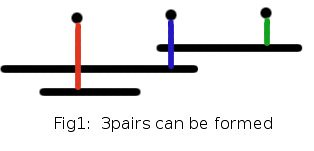# PACKBAND - Editorial

EASY

### EXPLANATION

This problem can be solved using a greedy strategy. We are given that a (R1,R2) rubber band can be used to pack a cubical box of side length L, only if 2 * 22/7 * R1 <= 4 * L <= 2 * 22/7 * R2. To avoid working with floating point numbers, we can multiply the equation with (7/4) on all sides and we get 11 * (R1) <= 7L <= 11 * (R2). Taking A = 11 * (R1), B = 11 * (R2) and C = 7 * L, we have A <= C <= B. So the problem is, given some intervals [Ai, Bi] (0 < = i < M ) and some points Cj ( 0 < = j < N), we can make a pair (intervali,pointj) if point j lies in the closed interval i. We need to make maximum number of (interval,point) pairs where each interval and point appears in at most one pair. This is like Maximum Bipartite matching. But the graph is not any arbitrary graph and has some properties, which can be used to design a more efficient algorithm. This new problem is very similar to the standard Interval Scheduling Problem, which has a nice greedy strategy ( Choose the earliest finishing time first ).We sort the points in ascending order and process them from left to right. We will try to assign an interval to the current point under consideration. If there are many intervals that can be assigned, which one to choose ? This is where the need of a good greedy strategy is. If we assign an interval which finish earliest ( i.e., smallest Bi), then there is a good chance that more intervals will be available for the points on the right. Even in the optimal assignment if we take the left most point that is paired with an interval which is not the earliest finishing interval, reassigning the point to the earliest finishing interval will not make the answer worse. This is just for intuitive understanding and a formal proof will be similar to that of the Interval Scheduling Problem.

{ I hope the reason why some of you couldn’t solve the problem is because you were not able to code while thinking of Samosa}

Exercise : Though we kept the constraint on N very low ( 1 <= N <= 1000 ) to allow O(N2) to pass, getting a simple O(N logN) is also easy. Think of how to solve this in O(N logN)

### SETTER’S SOLUTION

Can be found here.

### TESTER’S SOLUTION

Can be found here.

//